• ## lattice

千次阅读 2019-03-02 14:31:41
lattice 在实际的语音识别系统中，最优路径不一定与实际字序列匹配，我们一般希望能够得到得分最靠前的多条候选路径，即N-best。为了紧凑地保存候选路径，我们一般采用lattice(词图)来保存识别的候选序列。lattice...
lattice 在实际的语音识别系统中，最优路径不一定与实际字序列匹配，我们一般希望能够得到得分最靠前的多条候选路径，即N-best。为了紧凑地保存候选路径，我们一般采用lattice(词图)来保存识别的候选序列。lattice本质上是一个有向无环图。图上的每个节点代表一个词的结束时间点，每条边代表一个可能的词，以及该词发生的声学得分和语言模型得分。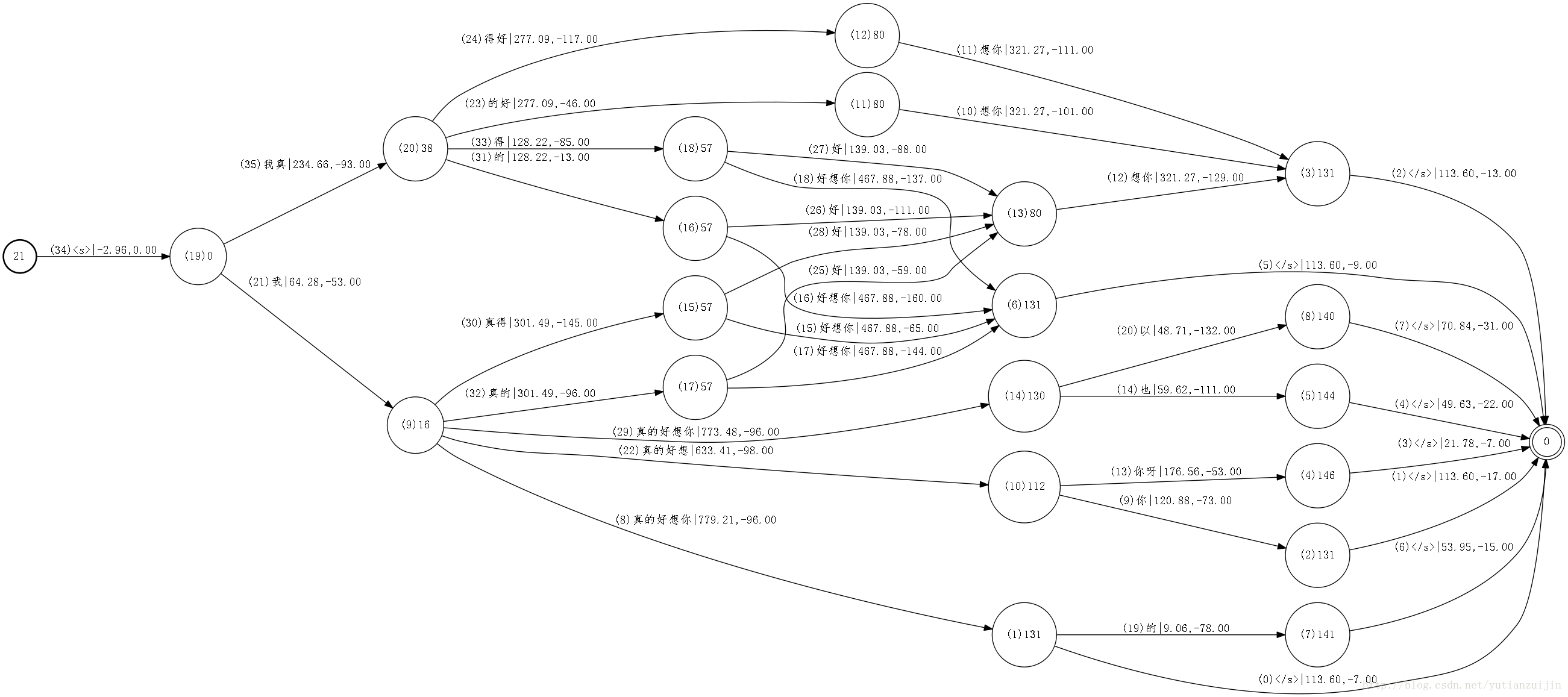在生成one-best结果时，只需要从最优结果处回溯，但是为了生成lattice，我们需要保留更多信息，具体就是生成每一个hist的时候保留多个候选alt，这样在回溯best hist的时候也访问alt来生成lattice。可以认为原始hist是有效信息再节点上的lattice，而上图中的lattice有效信息都在边上。在lattice上从左往右的任何一条路径，就构成一个识别结果，路径上每条边的声学得分相加，再加上路径对应的语言得分，就是整条路经的得分,通常取得分最大的前N条路径对应的词串作为识别的N-Best结果输出。上面lattice的生成用的语言模型往往不够精确，通常还需要在lattice上用更大的语言模型进行rescore。lattice剪枝 原始的lattice可能会非常庞大，上图给出的只是一个很小的lattice，这是我们可以对lattice进行剪枝但是不影响最终的准确率。一种剪枝方法是对lattice进行前向后向打分，计算每条边的后验概率，然后删除后验概率很低的边。对上图进行剪枝后获得下图：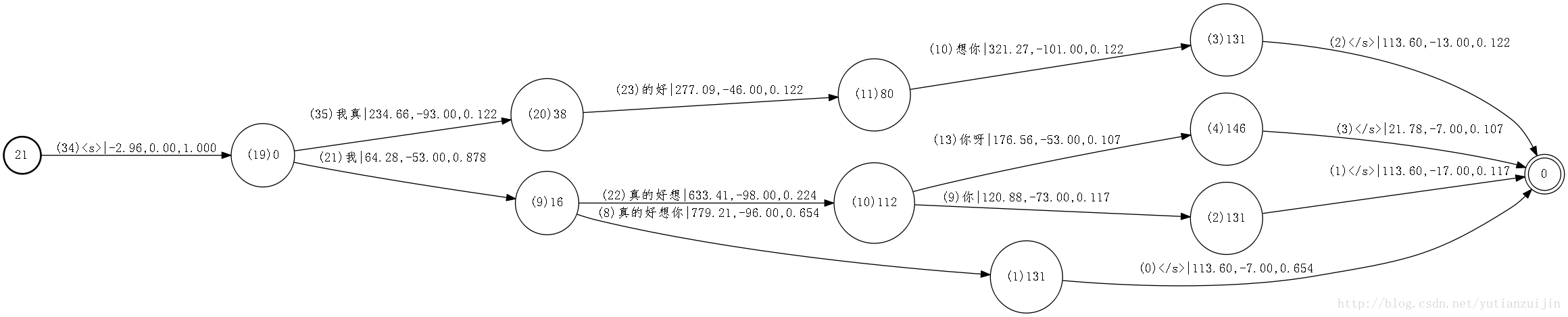相比原图，上面的lattice简化了不少，但是最重要的信息并没有丢失。通过计算后验概率，我们可以很容易地知道每条边在整个lattice中的重要性。 https://www.smwenku.com/a/5b83ca272b71777cb15c1388lattice操作中的函数 latgen-faster-mapped：生成lattice lattice-scale：对lattice权重缩放。 lattice-add-penalty:lattice中添加单词插入惩罚 lattice-best-path:通过lattice生成one-best路径，转录或者对齐作为输出。 lattice-align-words：转换lattice，使CompactLattice格式的弧与字词对应。 lattice-align-phones：转换lattices，使CompactLattice格式的弧与音素对应。
https://pages.harryfyodor.xyz/2017/11/13/decoder-intro/ 有了HCLG解码图，如何解码？ 首先，假设输入的语音有T帧，我们将之构建成T+1的状态WFSA。边上的信息是上下文相关的HMM模型和相关的loglikelihood。把这一个WFSA和已经有的HCLG进行合并得到一个图。这个图叫做搜索图，而解码的过程就相当于在这个搜索图里面寻找最优路径，可以使用维特比算法加上剪枝来寻找最优路径。 在kaldi实现中，合成的搜索图的边信息需要包括图损失（graph cost）和语音损失（acoustic cost）。实际操作中，对每一个HCLG的边，计算每一帧的语音损耗，每过25ms进行一次剪枝。最后得到一个lattice，记为P。把P输入输出翻转，得到Q。翻转的目的是为了对每个词序列里的词都保留最佳的路线(此时的Q输入是单词，输出是一个一个概率分布)，保留最好的分割方案。然后再编码为E。最后讲过一些优化，就可以得到最好的L。L的每一条路径都是当前语音输入的可能结果。
展开全文• Lattice是一个无环WFSA，结点可以是hmm状态、hmm(音素)、词，每个结点是一段音频在某个时间的对齐 用训练好的声学模型、现成的语言模型和发音字典构建解码网络(wfst)，最后将提取的测试集的语音特征输入以上解码...

Lattice是一个无环WFSA，结点可以是hmm状态、hmm(音素)、词，每个结点是一段音频在某个时间的对齐
用训练好的声学模型、现成的语言模型和发音字典构建解码网络(wfst)，最后将提取的测试集的语音特征输入以上解码网络，得到网格结构(lattice)的语音识别结果。
Lattice可用于语言模型的得分重估计
一个Lattice是为一段音频产生的，Lattice是一个带权无向图，其中每个点代表一个声学单元，每条弧包含两个权重(声学权重和语言权重)。解码时，会使用Viterbi算法?结合两种权重搜索Lattice，得分最高的路径就是解码结果。

识别的结果以lattice呈现，可以保存为standard lattice format (SLF)文件。
Lattice输出可以用于重打分、生成n-best结果.

Lattice类型只是一个基于特定半环模板化的 FST

来自 <https://shiweipku.gitbooks.io/chinese-doc-of-kaldi/content/lattice.html>

Lattice的运算操作：
修剪Lattice
计算最优路径
计算N-best结果
语言模型重打分
概率缩放
Lattice联合（用于MMI）
Lattice组合
Lattice内插

Lattice由LatticeSimpleDecoder类（解码器）生成，这个类是一个从SimpleDecoder类修改得来。 SimpleDecoder是Viterbi-Beam搜索算法的一种直接实现。
Lattice的产生过程大致为：LatticeSimpleDecoder首先产生状态级晶格，并且用Lattice-Delta修剪它，然后使用特殊的确定性算法，只保留每个单词序列的最佳路径。
http://kaldi-asr.org/doc/lattices.html

因为每个状态一个token，限制了可能得到的不同token历史记录的数量，所以他们不是最合适的。若每个模型状态对应多个token，并且如果认为来自不同前序单词的token是不同的，就可以避免上述限制。这样就为生成hypotheses（译者注：意为可能的识别结果）的lattice（译者注：网格）提供了可能，lattice比单Best输出更有用。基于这个思想的算法称为lattice N-best。
对输入语音进行解码转录成文本形成搜索空间， 为了减少识别错误，这种搜索空间通常不是单一的，而是以多个假设的形式存在（如网格形式, N-best 等）

Lattice
Lattices describe all theories considered by the Recognizer that have not been pruned out. Lattices are a directed graph containingNodes and Edges. A Node that corresponds to a theory that a word was spoken over a particular period of time. An Edge that corresponds to the score of one word following another. The usual result transcript is the sequence of Nodes though the Lattice with the best scoring path. Lattices are a useful tool for analyzing "alternate results".
Lattices是一个有向图，结点是词，边是转移概率。转录的结果是遍历Lattices后得到的最高得分词序列

源代码中Lattice类型的解析
typedef fst::VectorFst<LatticeArc> Lattice;
Lattice是一个弧为LatticeArc基于VectorFst模板的FST
typedef fst::ArcTpl<LatticeWeight> LatticeArc;
LatticeArc是一个弧参数为LatticeWeight基于ArcTpl的类
typedef fst::LatticeWeightTpl<BaseFloat> LatticeWeight;
LatticeWeight是一个使用BaseFloat类型作为浮点型基于LatticeWeightTpl的类
LatticeWeightTpl是一个模板,定义在fst命名空间(fstext/lattice-weight.h),与词典半环类似
即OpenFst中的LexicographicWeight模板:
LexicographicWeight<TropicalWeight, TropicalWeight>
这两个模板的加运算都被定义为取max，但是"max"被不同地定义。
LexicographicWeight首先比较第一个元素，并使用第二个元素打破关系。 LatticeWeight首先比较总和; 然后使用差异来打破关系。 因此，具有(a，b)的LatticeWeight等价于具有(a + b，a-b)的LexigraphicWeight。 LatticeWeight背后的基本直觉是保持采用最低代价路径(其中总代价是语言加声学代价)的语义，同时分别"记住"声学代价和语言代价是什么。 在理想世界中，我们可能希望保持更多的信息分离：例如，语言模型代价,转换模型代价,发音概率代价。 但这是不实际的，因为这些信息在解码图中全部混合在一起，并且在解码图中将其分离将导致解码图大小的显着扩展。

如前所述，Lattice中的输入符号表示transition-ids，输出符号表示字(或解码图的输出上的任何符号)。
当设计Kaldi时，我们考虑使用LexicographicWeight类型，而不是LatticeWeight类型，其中第一个元素是(graph + acoustic)成本，第二个元素就是声音成本。 我们最终决定反对这一点，因为虽然它可能为某些目的稍微更有效，我们认为这将是太混乱。
在lattice上的许多算法(例如，采取最佳路径或修剪)对于使用Lattice类型而不是CompactLattice类型是最有效的。 问题是，使用CompactLattice类型，权重包含transition-id的序列，并且像采取最佳路径的操作将权重作乘法，这对应于将这些序列链接在一起。 对于许多算法，需要的时间是lattice中字长度的两倍。如上所述，您可以从档案(ark)中读取Lattice，即使它包含CompactLattice，因为Holder类型(LatticeHolder)将执行自动转换。 因此，即使在"lats_rspecifier"中指定的归档文件或脚本文件包含CompactLattice格式(通常会这样)，下面代码仍然有效，因为这是我们通常写Lattice的方式。
...
}
格式类型转换的示例是：
Lattice lat;
// initialize lat.
CompactLattice compact_lat;
ConvertLattice(lat, &compact_lat);
转换为CompactLattice类型涉及一个"因式分解"操作，函数fst :: Factor()(由fstext / factor.h中的us定义)，它标识出可以组合成一个CompactLattice弧的状态链。 将OpenFst算法应用于lattice的典型示例如下(此代码，从latbin / lattice-best-path.cc修改，找到通过lattice的最佳路径)：
Lattice lat;
// initialize lat.
Lattice best_path;
fst::ShortestPath(lat, &best_path);

计算通过lattice的最佳路径
程序 lattice-best-path 计算通过lattice的最佳路径，并输出相应的输入符号序列(对齐,alignment)和输出符号序列(转录,transcription)。 像往常一样，输入和输出是以档案的形式。示例命令行是:
lattice-best-path --acoustic-scale=0.1 ark:in.lats ark:out.tra ark:out.ali
程序lattice-nbest计算通过lattice的N个最佳路径(使用OpenFst的ShortestPath()函数)，并将结果作为lattice(CompactLattice)输出，但其具有特殊结构。 如OpenFst中的ShortestPath()函数所记录的，开始状态有(最多)n个发射弧，每个弧连接到一条单独路径的开始。 注意，这些路径可以共享后缀。 示例命令行是：
lattice-nbest --n=10 --acoustic-scale=0.1 ark:in.lats ark:out.nbest
语言模型重打分
因为LatticeWeight的"图部分"（第一部分）包含的语言模型得分与transition-model得分和任意发音或静音概率混合在一起的，所以我们不能将其替换为新的语言模型得分，否则我们会失去转移概率和发音概率。 相反，我们必须先减去"旧"LM概率，然后添加新的LM概率。 这两个阶段的核心操作是"组合"（也有一些权重的缩放）。 这样做的命令行是：首先，删除旧的LM概率：
lattice-lmrescore --lm-scale=-1.0 ark:in.lats G_old.fst ark:nolm.lats
然后添加新的LM概率:
lattice-lmrescore --lm-scale=1.0 ark:nolm.lats G_new.fst ark:out.lats
注意，还有其他方法来做到这一点;请参阅下面的lattice-compose的文档(告诉我们程序lattice-lmrescore是什么).实际上，我们将首先使用简单的版本。假设我们给出了LM-scale和LM G.fst。我们首先将G.fst中的所有代价乘以S.然后对于每个lattice，我们在右边用G组成它(L o G)，对其使用lattice-determinize（仅保留每个单词序列通过G的最佳路径），并将其输出。这对positive S的效果良好，但对于negative S，它将相当于对每个单词序列采用通过G的最差路径。为了解决这个问题，我们做如下改动。对于每个输入的lattice，我们首先通过S的倒数来缩放点阵的语言模型（或LM）成本;我们然后在G.fst右边合成;我们对所得到的lattice运行lattice-determinize（参见上文），其对每个单词序列仅保留通过lattice的最佳路径;然后我们将lattice图/ LM分数缩放为S.这对于negative S是正确的。当输入的lattice对于每个单词序列仅具有通过它的单个路径时,上述过程才有意义（例如，如果它是lattice确定的）。我们假设在程序之间传递的所有lattice都有这个属性（这就是为什么写出"raw"状态级lattice不是一个好主意，除非你知道你在做什么）。

注意，为了使composition(组合的fst)工作，该程序需要将G.fst从"极小－加法半环"上映射到LatticeWeight半环。 这通过将G.fst的权重放入权重的第一部分（"图"部分），并且将权重的第二部分置为零（在半环中，这是1）来做到这一点。在C++级别，这个映射使用OpenFst的MapFst机制来完成.其中,我们定义了一个Mapper类来将StdArc映射到LatticeArc，然后创建一个类型为MapFst的对象，根据需要进行评估，并将G.fst转换为LatticeWeight权重类型。

lattice的改进

人们为了研究如何对ASR的输出建立有效的索引进行了
很多研究，lattice中包含大量的冗余信息，除了直接在lattice上建立索引之
外，人们还研究了如何去除lattice中的冗余信息。人们提出了类似lattice的
模型，但去除了lattice中的冗余信息，如混淆网络

和PSPLs(Position
SpecificPosteriorLattices)。转载于:https://www.cnblogs.com/JarvanWang/p/7499559.html
展开全文• ## Lattice LSTM

千次阅读 2018-12-17 20:30:24
Lattice LSTM Lattice LSTM来自于2018年发表在ACL的文章《Chinese NER Using Lattice LSTM》。这里只介绍 Lattice LSTM，论文其它内容不作介绍。 Lattice LSTM能够将字符级别序列信息和该序列对应的词信息同时编码供...
Lattice LSTM - 将分词信息带入LSTM
Lattice LSTM来自于2018年发表在ACL的文章《Chinese NER Using Lattice LSTM》。这里只介绍 Lattice LSTM，论文其它内容不作介绍。
Lattice LSTM能够将字符级别序列信息和该序列对应的词信息同时编码供模型自动取用。相较于字粒度（字符级）的编码，Lattice LSTM加入了词信息，丰富了语义表达；相较于词粒度的编码，Lattice LSTM可以避免分词错误带来的影响。
对于序列“南京市长江大桥“，每个位置的字粒度以及词粒度输入如下表所示：
当前位置（

j

j

）字粒度词粒度1南2京南京3市南京市4长市长5江长江6大7桥大桥、长江大桥
下图为Lattice LSTM处理字符序列（当前位置

j

j

没有对应的词序列，如上表位置1和6所示情况）的LSTM结构。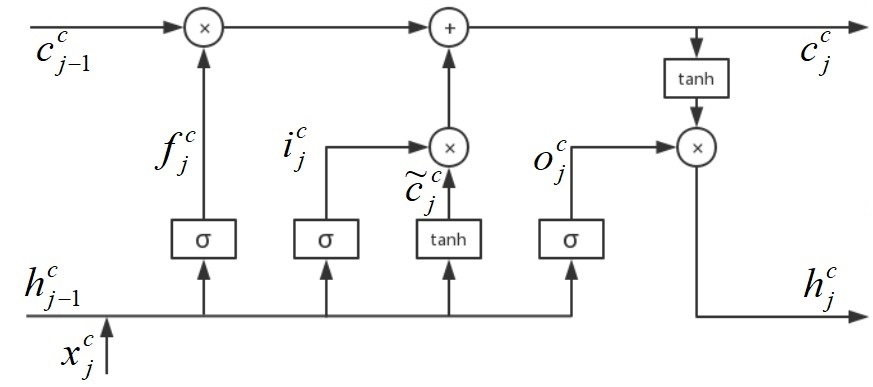其对应的数学公式（上标

c

c

表示字粒度变量，下标

j

j

表示序列的当前位置，

x

j

c

x_j^c

表示当前输入的字粒度信息）如下：

[

i

j

c

o

j

c

f

j

c

c

~

j

c

]

=

[

σ

σ

σ

t

a

n

h

]

(

W

c

⊤

[

x

j

c

h

j

−

1

c

]

+

b

c

)

c

j

c

=

f

j

c

⊙

c

j

−

1

c

+

i

j

c

⊙

c

~

j

c

h

j

c

=

o

j

c

⊙

t

a

n

h

(

c

j

c

)

\begin{aligned} \left[ \begin{array}{cccc} \textbf{i}^c_j\\ \textbf{o}^c_j\\ \textbf{f}^c_j\\ \widetilde{\textbf{c}}^c_j \end{array} \right ] &= \left[ \begin{array}{cccc} \sigma\\ \sigma\\ \sigma\\ tanh \end{array} \right ] \left ( \textbf{W}^{c\top} \left [ \begin{array}{cc} \textbf{x}^c_j\\ \textbf{h}^c_{j-1} \end{array} \right ] +\textbf{b}^c \right ) \\ \textbf{c}^c_j &= \textbf{f}^c_j \odot \textbf{c}^c_{j-1} + \textbf{i}^c_j \odot \widetilde{\textbf{c}}^c_j \\ \textbf{h}^c_j &= \textbf{o}^c_j \odot tanh(\textbf{c}^c_j) \end{aligned}

下图为Lattice LSTM处理词粒度输入的结构（注意，其

c

b

c

c_b^c

以及

h

b

c

h_b^c

使用的是

b

b

位置的字粒度隐状态

c

b

c

c_b^c

）：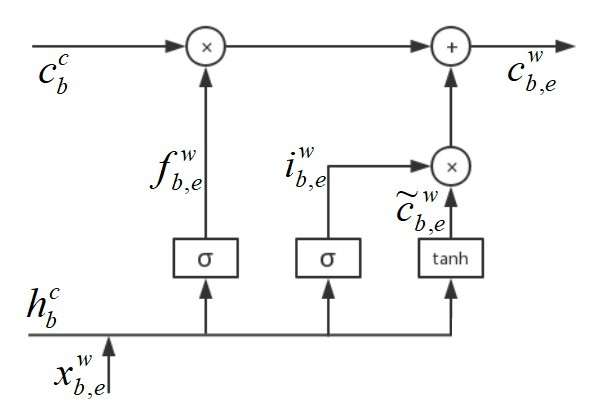其对应的数学公式（上标

w

w

表示词粒度变量，下标

b

b

和

e

e

分别表示词在序列中的开始与结束位置，

x

b

,

e

w

x_{b,e}^w

表示当前输入的词粒度信息）如下：

[

i

b

,

e

w

f

b

,

e

w

c

~

b

,

e

w

]

=

[

σ

σ

t

a

n

h

]

(

W

w

⊤

[

x

b

,

e

w

h

b

c

]

+

b

w

)

c

b

,

e

w

=

f

b

,

e

w

⊙

c

b

c

+

i

b

,

e

w

⊙

c

~

b

,

e

w

\begin{aligned} \left[ \begin{array}{ccc} \textbf{i}^w_{b,e}\\ \textbf{f}^w_{b,e}\\ \widetilde{\textbf{c}}^w_{b,e} \end{array} \right ] &= \left[ \begin{array}{ccc} \sigma\\ \sigma\\ tanh \end{array} \right ] \left ( \textbf{W}^{w\top} \left [ \begin{array}{cc} \textbf{x}^w_{b,e}\\ \textbf{h}^c_b \end{array} \right ] +\textbf{b}^w \right ) \\ \textbf{c}^w_{b,e} &= \textbf{f}^w_{b,e} \odot \textbf{c}^c_b + \textbf{i}^w_{b,e} \odot \widetilde{\textbf{c}}^w_{b,e} \end{aligned}

Lattice LSTM处理字符序列附加词信息（当前位置

j

j

i

b

,

e

c

=

σ

(

W

l

⊤

[

x

e

c

c

b

,

e

w

]

+

b

l

)

\textbf{i}^c_{b,e} = \sigma \left ( W^{l\top} \left[ \begin{array}{cc} \textbf{x}^c_e \\ \textbf{c}^w_{b,e} \end{array} \right ] + \textbf{b}^l \right )

于是，处理字粒度结构的隐状态

c

j

c

c_j^c

变化为以下计算形式：

c

j

c

=

∑

b

∈

{

b

′

∣

w

b

′

,

j

d

∈

D

}

α

b

,

j

c

⊙

c

b

,

j

w

+

α

j

c

⊙

c

~

j

c

\textbf{c}^c_j = \sum_{b \in \left\{ b'|w^d_{b',j} \in \mathbb{D} \right \}} \textbf{$\alpha$}^c_{b,j} \odot \textbf{c}^w_{b,j} + \textbf{$\alpha$}^c_j \odot \widetilde{\textbf{c}}^c_j

其中

α

b

,

j

c

\alpha_{b,j}^c

与

α

j

c

\alpha_j^c

的计算公式如下：

α

b

,

j

c

=

e

x

p

(

i

b

,

j

c

)

e

x

p

(

i

j

c

)

+

∑

b

′

∈

{

b

′

′

∣

w

b

′

′

,

j

d

∈

D

}

e

x

p

(

i

b

′

,

j

c

)

α

j

c

=

e

x

p

(

i

j

c

)

e

x

p

(

i

j

c

)

+

∑

b

′

∈

{

b

′

′

∣

w

b

′

′

,

j

d

∈

D

}

e

x

p

(

i

b

′

,

j

c

)

\begin{aligned} \textbf{$\alpha$}^c_{b,j} &= \frac{exp(\textbf{i}^c_{b,j})}{exp(\textbf{i}^c_j) + \sum_{b' \in \left\{ b''|w^d_{b'',j} \in \mathbb{D} \right \}}exp(\textbf{i}^c_{b',j})} \\ \textbf{$\alpha$}^c_j &= \frac{exp(\textbf{i}^c_{j})}{exp(\textbf{i}^c_j) + \sum_{b' \in \left\{ b''|w^d_{b'',j} \in \mathbb{D} \right \}}exp(\textbf{i}^c_{b',j})} \end{aligned}

由此可见，当当前位置

j

j

存在词粒度信息时，Lattice LSTM处理字粒度的结构的隐状态

c

j

c

c_j^c

会发生变化，不再是字粒度序列的信息，而是来自词粒度的信息。在信息往下传递时，隐状态

c

j

c

c_j^c

用学到的词粒度的信息替换掉了来自字粒度的信息。当然，这并不意味着将当前位置之前所有字粒度信息都丢弃了，因为新的隐状态

c

j

c

c_j^c

中使用了

c

~

j

c

\widetilde{c}_j^c

，保留了来自词在序列中开始位置之前的字粒度信息。所以，这里完全不需要考虑遗忘门没有使用的问题（参考2中有提到）。
另外，

h

j

c

h_j^c

的计算仍采用原来的方式计算。猜测原因是当前位置之前的词长度的序列的结果以及隐状态已经确定，无法使用当前位置的词粒度信息。所以，作者提出的Lattice LSTM只能让词粒度信息影响当前位置后面的序列，并不能对当前位置的结果进行直接影响。鉴于此，实际使用中可以考虑双向结构，即类似于Bi-LSTM这样的结构，或者设计一个门结合之前的各位置状态信息更改之前位置的预测结果。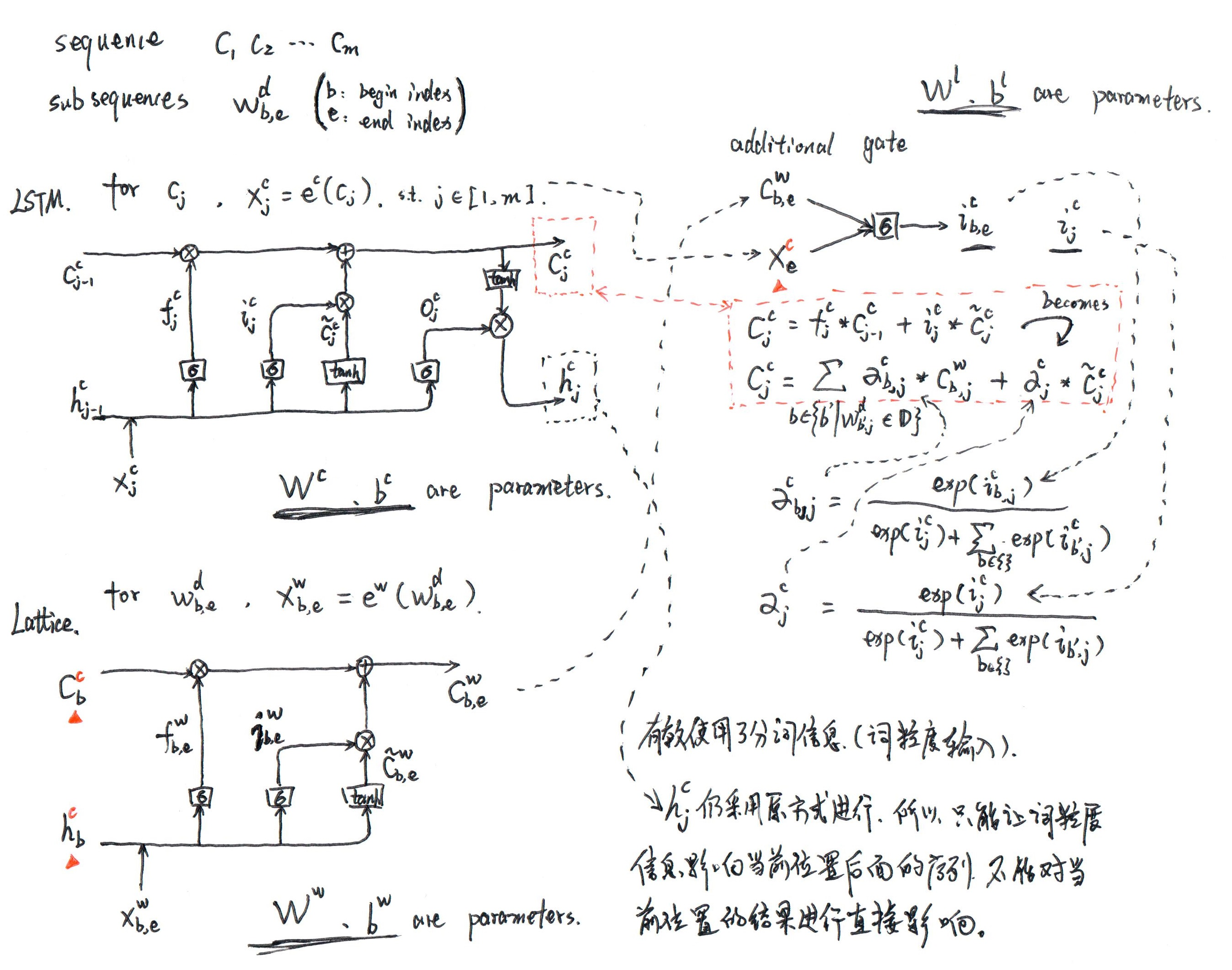总结，该结构有效的使用了分词信息（词粒度输入），考虑到其在实现上相较于传统的LSTM结构更复杂，可以考虑采用其它方式将分词信息带入，例如模型的输入可以多一个分词特征向量。
参考
1. paper notes 《Chinese NER Using Lattice LSTM》
2. 论文阅读总结——Chinese NER Using Lattice LSTM
展开全文LSTM acl 神经网络
• LATTICE Diamond 3.11 Make license Gen
• Lattice FPGA 开发软件 Diamond License，亲测支持V3.07, V3.09, V3.10;
• kaldi lattice


概况
两种lattice结构
Lattice结构
FST的形式，weight包括两部分（graph cost和acoustic cost），输入是transition-ids，输出是words。 其中weight的graph cost包含LM+transition+pronunciation三部分。
CompactLattice结构
和lattice相似，区别在于它是接收机，输入和输出一样（都是words），weight包含两部分（权重和transition-ids），相比于Lattice，CompactLattice把输入的transition-ids转移到weight上面。
lattice保证每一个word-sequence只对应lattice中的一条路径。
Lattice实现
假设a表示graph cost，b表示acoustic cost，那么 LatticeWeight表示为(a,b) LexicographicWeight表示为(a+b,a-b)
lattice相关的算法在Lattice上面更为高效，因为CompactLattice的weight包含有transition-ids，当take best path的时候就涉及到transition-ids的拼接操作。
lattice写入文件时保持acoustic cost是unscaled。可以从archive中读取Lattice，即使里面包含有CompactLattice。
可以使用ConvertLattice()函数将Lattice转化为CompactLattice。lattice转化：
  Lattice lat;  // initialize lat.  CompactLattice compact_lat;  ConvertLattice(lat, &compact_lat);
1234
使用OpenFst算法
  Lattice lat;  // initialize lat.  Lattice best_path;  fst::ShortestPath(lat, &best_path);
1234
CompactLattice实现
lattice generation
对应的类 LatticeSimpleDecoder，流程包括： - 产生state级别的lattice - 使用lattice-delta对lattice剪枝 - 使用特殊的确定化算法对每一个word sequence只保留最优路径
lattice operation
lattice-prune --acoustic-scale=0.1 --beam=5 ark:in.lats ark:out.latslattice-best-path --acoustic-scale=0.1 ark:in.lats ark:out.tra ark:out.alilattice-nbest --n=10 --acoustic-scale=0.1 ark:in.lats ark:out.nbest#LM rescorelattice-lmrescore --lm-scale=-1.0 ark:in.lats G_old.fst ark:nolm.latslattice-lmrescore --lm-scale=1.0 ark:nolm.lats G_new.fst ark:out.lats#probability scalinglattice-scale --acoustic-scale=0.1 --lm-scale=0.8 ark:in.lats ark:out.lats#用在MMI的区分度训练MMI，保证正确的transcription出现在分母lattice-union ark:num_lats.ark ark:den_lats.ark ark:augmented_den_lats.arklattice-interp --alpha=0.4 ark:1.lats ark:2.lats ark:3.latslattice-to-phones final.mdl ark:1.lats ark:phones.latslattice-project ark:1.lats ark:- | lattice-compose ark:- ark:2.lats ark:3.latslattice-equivalent ark:1.lats ark:2.lats || echo "Not equivalent!"lattice-rmali ark:in.lats ark:word.lats#Boosted MMI traininglattice-boost-ali --silence-phones=1:2:3 --b=0.1 final.mdl ark:1.lats \   ark:1.ali ark:boosted.lats#前后向计算后验概率lattice-to-post --acoustic-scale=0.1 ark:1.lats ark:- | \    gmm-acc-stats 10.mdl "$feats" ark:- 1.acclattice-determinize ark:1.lats ark:det.lats#计算WER cat$data/text | \  sed 's:<NOISE>::g' |  sed 's:<SPOKEN_NOISE>::g'  | \  scripts/sym2int.pl --ignore-first-field $lang/words.txt | \ lattice-oracle --word-symbol-table=$lang/words.txt  \    "ark:gunzip -c $dir/lats.pruned.gz|" ark:- ark,t:$dir/oracle.tra \    2>\$dir/oracle.log#增加转移概率lattice-add-trans-probs --transition-scale=1.0 --self-loop-scale=0.1 \      final.mdl ark:1.lats ark:2.latslattice-to-fst --lm-scale=0.0 --acoustic-scale=0.0 ark:1.lats ark:1.wordslattice-copy ark:1.lats ark,t:- | head -50lattice-to-nbest --acoustic-scale=0.1 --n=10 ark:1.lats ark:1.nbestnbest-to-linear ark:1.nbest ark:1.ali ark:1.words ark:1.lmscore ark:1.acscore
12345678910111213141516171819202122232425262728293031323334353637
对于lattice来讲，边上的word/transition-id/weight并不是完全的对应关系，同时得到的时间信息也是不准确的. 对于CompactLattice来说，单个边上的信息意义不明确，只有组合成一条完整的path才有意义。

展开全文• lattice低功耗低成本FPGA ICE40UP5K资料合集。收集于官网。
•代码
• lattice基本教程，很实用，入门基本指导 ，其中包含使用vorilog建立工程，下载，并调试的整个过程，图文并茂
• Lattice Semiconductor(莱迪思)发表采用90nm制程的第三代非挥发性(NV)FPGA系列LatticeXP2，强调通过最新的True NV FPGA技术，可提供弹性化逻辑、最小占位空间、高度安全性，以及低于1ms的瞬时激活(instant-on)等优势...
• lattice diamond 3.7 license 莱迪思(Lattice) 亲测保证可用
• 1 lattice和ali.gz区别 【产生的lattice文件】 是ASR转写的候选结果路径的存储（简单理解就是ASR转写的候选结果），将所有候选路径都存在了这个lattice中，就可以在这个候选结果中去挑选最佳的候选结果(即one best...
• grid.js grid.js主页 网址： ： 在本地运行主页 主页的内容存储在docs目录中，并从main分支提供服务。 已设置NPM和webpack，以便在使用webpack服务器在本地运行NPM并编辑页面时，该页面将被热重新加载。... latticeJavaScript
• Lattice 1. some definitions real space lattice, $$\overrightarrow{a_1}$$, $$\overrightarrow{a_2}$$, $$\overrightarrow{a_3}$$; reciprocal space lattice, reclat, $$\overrightarrow{rec_1}$$, \(\over...
• Lattice gas cellular automata and lattice Boltzmann models
• Lattice animal is a set of connected sites on a lattice. Lattice animals on a square lattice are especially popular subject of study and are also known as polyominoes. Polyomino is usually represented...as numbers
•Shell

千次下载 热门讨论 2013-07-15 23:04:45...